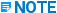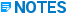# US Code Stresses - CAESAR II - Installation & Upgrade

## CAESAR II Quick Reference

Language
English
Product
CAESAR II
Search by Category
CAESAR II Version
13

The US code-based stress equations and load case labels used by CAESAR II for actual and allowable stresses are shown below.

The load case recommendations made by CAESAR II are usually sufficient for code compliance. CAESAR II does not recommend occasional load cases. Occasional loads are unknown in origin, and you must specify them.

Code Equation

Allowable

Longitudinal Pressure Stress - Slp

Slp = PDo / 4tn

Code approximation

Slp = PDi2 / (Do2 - Di2)

Code exact equation (CAESAR II Default)

Operating Stress – unless otherwise specified

S = Slp + Fax/Am + Sb

N/A

B31.1 (2018 Edition)

Sl = Slp + 0.75iMa / Z

< Sh

SUS

Se = iMc / Z

< f[1.25(Sc + Sh) - Sl]

EXP

Slp + 0.75iMa / Z + 0.75iMb / Z

< kSh

OCC

B31.1 (2020 Edition) (Requires B31J)

Sl = [(|Slp + IaFax / Am| + Sb)2 + 4St2]1/2

Where:
Sb = [(IiMi)2 + (IoMo)2]1/2 / Z
St = ItMt / 2Z
Z is the nominal section modulus

≤ Sh

≤ kSh

SUS

OCC

Se = [(|iaFax / Am| + Sb)2 + 4St2 ]1/2

Where:
Sb = [(iiMi)2 + (ioMo)2]1/2 / Z
St = itMt / 2Z
Z is the nominal section modulus

≤ f [1.25(Sc + Sh) - Sl]

EXP

B31.3

SL = [(Ia (|Slp + Fax / Ap|) +Sb)2 + 4St2]1/2

Where:
Sb = [(liMi)2 + (loMo)2]1/2 / Z
Z is the corroded section modulusFor reduced outlet connections,
use Ze instead of Z if you do not specify
bending in/out-plane SIFs (ii and io) or
index (li and lo). Use Z if you specify
bending in/out-of-plane SIFs or index.

St = ltMt / 2Z

< Sh

< 1.33Sh

SUS

OCC

Se = [(|iaFax/Ap| +Sb)2 + 4St2]1/2

Where:

Sb = [(iiMi)2 + (ioMo)2]1/2 / Z

Z is the section modulus computed
from nominal dimensions (not corroded).For reduced outlet connections,
use Ze instead of Z if you do not specify
bending in/out-plane SIFs (ii and io) or
index (li and lo). Use Z if you specify
bending in/out-of-plane SIFs or index.

St = itMt / 2Z

< f [1.25(Sc + Sh) - SL]

EXP

B31.3 Chapter IX

SL = [(Ia (|Slp + Fax / Ap|) +Sb)2 + 4St2]1/2

Where:
Sb = [(liMi)2 + (loMo)2]1/2 / Z
Z is the corroded section modulusFor reduced outlet connections,
use Ze instead of Z if you do not specify
bending in/out-plane SIFs (ii and io) or
index (li and lo). Use Z if you specify
bending in/out-of-plane SIFs or index.

St = ltMt / 2Z

< Sh

< 1.2Sh

SUS

OCC

Se = [(ia (|Slp + Fax / Ap|) +Sb)2 + 4St2]1/2

Sb = [(iiMi)2 + (ioMo)2]1/2 / Z

Where:
Z is the section modulus computed
from nominal dimensions (not corroded).For reduced outlet connections,
use Ze instead of Z if you do not specify
bending in/out-plane SIFs (ii and io) or
index (li and lo). Use Z if you specify
bending in/out-of-plane SIFs or index.

St = itMt / 2Z

< 1.25Sc + 0.25 Sh

EXP

B31.4

Fully Restrained Pipe (B31.4/R)

Hoop:
Shoop = Pi D/2t (for D/t ³ 20)

or

Shoop = Pi (D-t)/2t (for D/t < 20)

< 0.72 ESy
(where E is the weld joint factor)

SUS, OPE, OCC

Expansion: Se = Ea(T1 − T2)

< 0.9 Sy

EXP

Longitudinal:
SL = Se + nSH + Sb+ Fa/A
where
Sb = [(Mi2 + Mo2)1/2]/Z

< 0.9 Sy

SUS, OPE, OCC

Equivalent Combined:
Seq = [(SL − SH)2 + 4St2]1/2

or

Seq = (SH2 − SHSL + SL2 + 3St2)1/2
where
St = Mt/2Z

< 0.9 Sy

SUS, OCC, OPE

Fully Above Ground, Unrestrained Pipe (B31.4/U)

Hoop:
Shoop = Pi D/2t (for D/t ³ 20)

or

Shoop = Pi (D-t)/2t (for D/t < 20)

< 0.72 ESy
(where E is the weld joint factor)

<0.90Sy when defined as Hydro (HYD)

SUS, OCC, HYD

Expansion:
Se = (Sb2 + 4St2)1/2
where
Sb = {[(iiMi)2 + (ioMo)2]1/2}/Z
St = Mt/2Z

f[1.25(Sc + Sh) - SL]Sc and Sh are 2/3Sy.

EXP

Longitudinal:
SL = PiD/4t + Sb + Fa/A
where
Sb = {[(iiMi)2 + (ioMo)2]1/2}/Z

< .75Sy

< .80Sy when defined as Occasional (OCC)

SUS, OCC, HYD

Equivalent Combined

Not used

Riser and Platform for Inland Waterways (B31.4/W)

Hoop:
Shoop = Pi D/2t (for D/t ³ 20)

or

Shoop = Pi (D-t)/2t (for D/t < 20)

< 0.6 ESy
(where E is the weld joint factor)

<0.90Sy when defined as Hydro (HYD)

SUS, OCC, HYD

Expansion:
Se = (Sb2 + 4St2)1/2
where
Sb = {[(iiMi)2 + (ioMo)2]1/2}/Z
St = Mt/2Z

< 0.8 Sy

EXP

Longitudinal:
SL = PiD/4t + Sb + Fa/A
where
Sb = {[(iiMi)2 + (ioMo)2]1/2}/Z

< 0.8 Sy

< 0.90Sy when defined as Occasional (OCC)

SUS, OCC, HYD

Equivalent Combined

Not usedWhen more than one stress evaluation is used, such as checking both hoop stress and longitudinal stress, CAESAR II reports the stress pair producing the largest calculated stress/allowable stress ratio.

B31.4 Chapter IX (Offshore)

Hoop:

Shoop = (Pi – Pe )D/2t (for D/t ³ 30)

< F1Sy

OPE, SUS, OCC

or

Shoop = (Pi – Pe )(D-t)/2t (for D/t < 30)

< 0.9Sy

HYD

Longitudinal:

SL = Slp + Sb +Fa/A

< 0.8Sy

OPE, SUS, OCC

where
Slp = (PiRi2 - PeRo2)/(Ro2 - Ri2)
Sb = [(iiMi)2 + (ioMo)2]1/2/Z

< 0.9Sy

HYD

Equivalent Combined:

Seq = [(SL – SH)2 + 4St2]1/2

or

Seq = (SH2 - SHSL + SL2 + 3St2)1/2
where
St = Mt/2Z

< 0.9Sy

OPE, SUS, OCC, HYDWhen more than one stress evaluation is used, such as checking both hoop stress and longitudinal stress, CAESAR II reports the stress pair producing the largest calculated stress/allowable stress ratio.

B31.4 Chapter XI (Slurry Pipes)

Fully Restrained Pipe (B31.4 Ch XI/R)

Hoop:
Shoop = Pi D/2t (for D/t ³ 20)

or

Shoop = Pi (D-t)/2t (for D/t < 20)

< 0.80 ESy
(where E is the weld joint factor)

SUS, OCC, OPE

Expansion:
Se = Ea(T1 − T2)

< 0.9 Sy

EXP

Longitudinal:
SL = Se + nSH + Sb+ Fa/A
where
Sb = [(Mi2 + Mo2)1/2]/Z

< 0.9 Sy

< 0.88 Sy when defined as Occasional (OCC)

SUS, OPE, OCC

Equivalent Combined:
Seq = [(SL − SH)2 + 4St2]1/2

or

Seq = (SH2 − SHSL + SL2 + 3St2)1/2
where
St = Mt/2Z

< 0.9 Sy

SUS, OCC, OPE

Fully Above Ground, Unrestrained Pipe (B31.4 Ch XI/U)

Hoop:
Shoop = Pi D/2t (for D/t ³ 20)

or

Shoop = Pi (D-t)/2t (for D/t < 20)

< 0.80 ESy
(where E is the weld joint factor)

<0.90Sy when defined as Hydro (HYD)

SUS, OCC, HYD

Expansion:
Se = (Sb2 + 4St2)1/2
where
Sb = {[(iiMi)2 + (ioMo)2]1/2}/Z
St = Mt/2Z

f[1.25(Sc + Sh) - SL]Sc and Sh are 2/3Sy

EXP

Longitudinal:
SL = PiD/4t + Sb + Fa/A
where
Sb = {[(iiMi)2 + (ioMo)2]1/2}/Z

< .75Sy

< .88Sy when defined as occasional (OCC)

SUS, OCC, HYD

Equivalent Combined

Not usedWhen more than one stress evaluation is used, such as checking both hoop stress and longitudinal stress, CAESAR II reports the stress pair producing the largest calculated stress/allowable stress ratio.

B31.5

Sl = Slp + Fax/Am +Sb

< Sh

SUS

(Sb2 + 4St2)1/2

< f[1.25(Sc + Sh) – Sl]

EXP

Fax/Am + Sb + Slp

< kSh

OCC

Sb = {[(iiMi)2 + (ioMo)2]1/2} / Z

B31.8 (2018 and 2020 Editions)

Restrained Pipe

Longitudinal:

SL = Slp + Sb + Sa

< 0.9TSy

SUS, OPE, OCCCAESAR II includes the thermal effect in Sa

Equivalent Combined:

Seq = max[ |Shoop - SL| , |Shoop| , |SL| ]

< 0.9TSy

SUS, OPE

< kTSy

OCC

or

Seq = (SL2 - SLShoop + Shoop2)1/2

< 0.9TSy

SUS, OPE

< kTSy

OCCThe equivalent combined stress is valid for straight sections of pipe only.

Unrestrained Pipe

Longitudinal:

SL = Slp + Sb + Sa

< 0.75TSy

SUS, OCC

< 0.75Sy

HYD

Expansion:

Se = ME/Z

< f[1.25(Sc + Sh) - SL]

where
Sc = 0.33SU
Sh = 0.33TSU

EXP

Where:

Shoop = PDo/2t

Slp = 0.3Shoop

Slp = 0.5Shoop

Restrained Pipe

Unrestrained Pipe

Sa = Fax/Am

Mb = [(0.75iiMi)2+(0.75ioMo)2]1/2

MR = [(0.75iiMi)2+(0.75ioMo)2 + Mt2]1/2

Sb = Mb/Z

Sb = MR/Z

Straight pipe

Fittings and components

ME = [(iiMi)2+(ioMo)2 + Mt2]1/2

B31.8 Chapter VIII (Offshore) (2018 and 2020 Editions)

Hoop Stress:

Sh = (Pi – Pe)D/2t, when D/t ³ 30

Sh = (Pi – Pe)(D – t)/2t, when D/t < 30

< F1SyT

OPE, SUS, OCC

Longitudinal Stress: |SL|

SL = Slp + Fax/Am ± Sb

Where:

Slp = (Pi * Ri2 - Pe * Ro2) / (Ro2 - Ri2)

Sb = {[(iiMi)2 + (ioMo)2]1/2}/Z

< 0.8Sy

OPE, SUS, OCC

Equivalent Combined Stress (Tresca) (2018 edition):

Seq = 2{[(SL – Sh)/2]2 + St2}1/2

< 0.9Sy

OPE, SUS, OCC

Equivalent Combined (Tresca) (2020 edition):

Seq = Maximum of absolute values of:

2{[(SL - Sh)/2]2 + St2}1/2
(SL + Sh)/2 - {[(SL - Sh)/2]2 + St2}1/2
(SL + Sh)/2 + {[(SL - Sh)/2]2 + St2}1/2

< 0.9Sy

OPE, SUS, OCC

Alternative Combined Stress (Von Mises)
(2018 and 2020 editions):

Seq = (Sh2 – SLSh + SL2 + 3St2)1/2

< 0.9Sy

Where:

F1 = Hoop Stress Design Factor (Table A842.2.2-1)
T = Temperature Derating Factor (Table 841.1.8-1)
Sy = Specified Minimum Yield Strength• For information on F1, T, and Sy, see Operating, sustained, and occasional load cases in the B31.8 Chapter VIII (Offshore) technical discussion.

• For the equivalent combined stress on platform piping and risers, B31.8 Chapter VIII bases the calculation of the stress components on the minimum corroded wall thickness. Pipelines use the nominal wall thickness.

B31.9

Paragraph 919.4.1.b states that analysis uses the equations of B31.1.

ASME SECT III CLASS 2 & 3

Sl =[B1PmaxDo / 2tn] + B2Ma / Z

< 1.5Sh

SUS

Se =iMc/Z

< f(1.25Sc + 0.25Sh) + (Sh - Sl)

EXP

B1Slpmax + B2(Ma + Mb) / Z

< 1.8Sh and < 1.5Sy

OCC

B31.1 (1967) and Navy Section 505

Sl = Slp + (Sb2 + 4St2)1/2

< Sh

SUS

Se = (Sb2 + 4St2)1/2

< f[1.25Sc + 0.25Sh +(Sh – Sl)]

EXP

Slp + (Sb2 + 4St2)1/2

< kSh

OCC

GPTC

Slp + 0.75iMa / Z

< Sy

OPE

Slp + Sb

< 0.75SyFt

SUS

(Sb2 + 4St2)1/2

< 0.72Sy

EXP